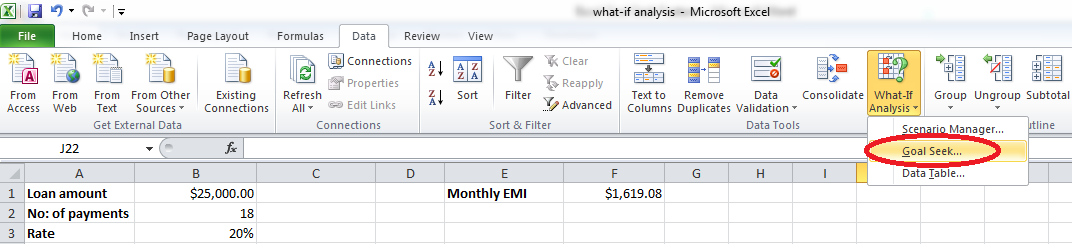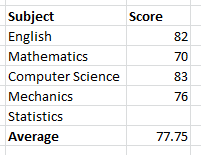### How To Do What-If Analysis Within Excel

What-If analysis is the process of calculating backward to find out an input by providing a specific output. In other words, what-if analysis can be considered as opposite to formulas. You use formulas to calculate an output by providing inputs whereas what-if analysis helps you find out what input will result in a specific output. You can use what-if analysis to estimate your monthly savings that meet your retirement goals, find the return on investment, plan your budget and so on.

There are three different tools available in Excel to perform what-if analysis: scenarios, data tables and Goal Seek. Let’s have a look at Goal Seek function. Using Goal Seek function, you can change the data in formula and analyze how it affects the end result. Have a look at the data given below (don’t get scared). We will be using this data to perform what-if analysis.

### Example 1 Monthly EMI calculation

Suppose a person takes a loan for \$25,000 from a bank at the interest rate of 20% per annum and he agrees to pay off the loan in one and a half years (18 months). All remaining data including monthly EMI, monthly payment towards principal, monthly payment towards interest and remaining amount to be paid each month are calculated using financial formulas.In this case, the person has to pay an amount of \$1619.08 every month for 18 months to complete the loan. Assume that the person is capable of paying \$2500 every month so that he can pay off the loan faster. So, how can we find out in how many months the person will be able to complete the loan if he pays \$2500 monthly. Here comes Goal Seek function into picture.

### Perform what-if analysis

Open Excel, copy the data shown above and save your file as what-if analysis.xlsx. Go to Data (main menu) –> What-If Analysis (in the Data Tools group) and select Goal Seek.You will get a window like this:Click in the textbox next to Set cell and click the cell E1 as we need to analyze the result by changing the monthly payment. In the To value: textbox, enter 2500 as this is our new value. After clicking in the By changing cell: textbox, click the cell B2 as we want to find out the change in number of payments (number of months). Now your window will look like this:Click OK and now your screen will look like this:If you analyze the value in cell B2, you could find that it is 11.03. It means that you can complete the loan in less than a year (in 11.03 months) if you make a payment of \$2,500 per month.

### Example 2 Notes average calculations

Suppose you are a student who plans to score an average of 80 in your semester exam. You scored 82, 70, 83 and 76 in the subjects English, Mathematics, Computer Science and Mechanics respectively. You have Statistics exam remaining and you want to calculate the marks you need to score in Statistics to achieve an average of 80. Your score sheet before the Statistics exam will be as follows:Select the Goal Seek function to open the window. Click in the textbox next to Set cell: and click the cell that contains the average score (here the cell containing the value 77.5). In the To value: textbox, enter 80 as this is your target average. After clicking in the By changing cell textbox, click the cell that will contain the score of Statistics (here the cell just above the cell with 77.5). Click OK and your screen will look like this:From this data it is clear that you have to score 89 in Statistics to achieve an overall average of 80. Similarly, you can use Goal Seek function in many different scenarios to analyze what input should be given in order to get a specific output.

## Template

```Further reading: